Hypothesis Testing with One Sample

# Distribution Needed for Hypothesis Testing

Earlier in the course, we discussed sampling distributions. Particular distributions are associated with hypothesis testing. Perform tests of a population mean using a normal distribution or a Student’s t-distribution. (Remember, use a Student’s t-distribution when the population standard deviation is unknown and the distribution of the sample mean is approximately normal.) We perform tests of a population proportion using a normal distribution (usually n is large).

If you are testing a single population mean, the distribution for the test is for means: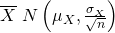or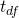The population parameter is μ. The estimated value (point estimate) for μ is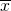, the sample mean.

If you are testing a single population proportion, the distribution for the test is for proportions or percentages: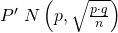The population parameter is p. The estimated value (point estimate) for p is p′. p′ =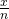where x is the number of successes and n is the sample size.

### Assumptions

When you perform a hypothesis test of a single population mean μ using a Student’s t-distribution (often called a t-test), there are fundamental assumptions that need to be met in order for the test to work properly. Your data should be a simple random sample that comes from a population that is approximately normally distributed. You use the sample standard deviation to approximate the population standard deviation. (Note that if the sample size is sufficiently large, a t-test will work even if the population is not approximately normally distributed).

When you perform a hypothesis test of a single population mean μ using a normal distribution (often called a z-test), you take a simple random sample from the population. The population you are testing is normally distributed or your sample size is sufficiently large. You know the value of the population standard deviation which, in reality, is rarely known.

When you perform a hypothesis test of a single population proportion p, you take a simple random sample from the population. You must meet the conditions for a binomial distribution which are: there are a certain number n of independent trials, the outcomes of any trial are success or failure, and each trial has the same probability of a success p. The shape of the binomial distribution needs to be similar to the shape of the normal distribution. To ensure this, the quantities np and nq must both be greater than five (np > 5 and nq > 5). Then the binomial distribution of a sample (estimated) proportion can be approximated by the normal distribution with μ = p and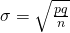. Remember that q = 1 – p.

### Chapter Review

In order for a hypothesis test’s results to be generalized to a population, certain requirements must be satisfied.

When testing for a single population mean:

1. A Student’s t-test should be used if the data come from a simple, random sample and the population is approximately normally distributed, or the sample size is large, with an unknown standard deviation.
2. The normal test will work if the data come from a simple, random sample and the population is approximately normally distributed, or the sample size is large, with a known standard deviation.

When testing a single population proportion use a normal test for a single population proportion if the data comes from a simple, random sample, fill the requirements for a binomial distribution, and the mean number of success and the mean number of failures satisfy the conditions: np > 5 and nq > n where n is the sample size, p is the probability of a success, and q is the probability of a failure.

### Formula Review

If there is no given preconceived α, then use α = 0.05.

Types of Hypothesis Tests
• Single population mean, known population variance (or standard deviation): Normal test.
• Single population mean, unknown population variance (or standard deviation): Student’s t-test.
• Single population proportion: Normal test.
• For a single population mean, we may use a normal distribution with the following mean and standard deviation. Means: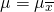and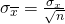• A single population proportion, we may use a normal distribution with the following mean and standard deviation. Proportions: µ = p and.

Which two distributions can you use for hypothesis testing for this chapter?

A normal distribution or a Student’s t-distribution

Which distribution do you use when you are testing a population mean and the population standard deviation is known? Assume a normal distribution, with n ≥ 30.

Which distribution do you use when the standard deviation is not known and you are testing one population mean? Assume sample size is large.

Use a Student’s t-distribution

A population mean is 13. The sample mean is 12.8, and the sample standard deviation is two. The sample size is 20. What distribution should you use to perform a hypothesis test? Assume the underlying population is normal.

A population has a mean is 25 and a standard deviation of five. The sample mean is 24, and the sample size is 108. What distribution should you use to perform a hypothesis test?

a normal distribution for a single population mean

It is thought that 42% of respondents in a taste test would prefer Brand A. In a particular test of 100 people, 39% preferred Brand A. What distribution should you use to perform a hypothesis test?

You are performing a hypothesis test of a single population mean using a Student’s t-distribution. What must you assume about the distribution of the data?

It must be approximately normally distributed.

You are performing a hypothesis test of a single population mean using a Student’s t-distribution. The data are not from a simple random sample. Can you accurately perform the hypothesis test?

You are performing a hypothesis test of a single population proportion. What must be true about the quantities of np and nq?

They must both be greater than five.

You are performing a hypothesis test of a single population proportion. You find out that np is less than five. What must you do to be able to perform a valid hypothesis test?

You are performing a hypothesis test of a single population proportion. The data come from which distribution?

binomial distribution

### Homework

It is believed that Lake Tahoe Community College (LTCC) Intermediate Algebra students get less than seven hours of sleep per night, on average. A survey of 22 LTCC Intermediate Algebra students generated a mean of 7.24 hours with a standard deviation of 1.93 hours. At a level of significance of 5%, do LTCC Intermediate Algebra students get less than seven hours of sleep per night, on average? The distribution to be used for this test is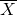~ ________________

1.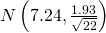2.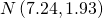3. t22
4. t21

d

### Glossary

Binomial Distribution
a discrete random variable (RV) that arises from Bernoulli trials. There are a fixed number, n, of independent trials. “Independent” means that the result of any trial (for example, trial 1) does not affect the results of the following trials, and all trials are conducted under the same conditions. Under these circumstances the binomial RV Χ is defined as the number of successes in n trials. The notation is: X ~ B(n, p) μ = np and the standard deviation is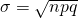. The probability of exactly x successes in n trials is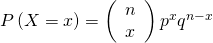.
Normal Distribution
a continuous random variable (RV) with pdf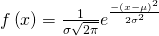, where μ is the mean of the distribution, and σ is the standard deviation, notation: X ~ N(μ, σ). If μ = 0 and σ = 1, the RV is called the standard normal distribution.
Standard Deviation
a number that is equal to the square root of the variance and measures how far data values are from their mean; notation: s for sample standard deviation and σ for population standard deviation.
Student’s t-Distribution
investigated and reported by William S. Gossett in 1908 and published under the pseudonym Student. The major characteristics of the random variable (RV) are:

• It is continuous and assumes any real values.
• The pdf is symmetrical about its mean of zero. However, it is more spread out and flatter at the apex than the normal distribution.
• It approaches the standard normal distribution as n gets larger.
• There is a “family” of t distributions: every representative of the family is completely defined by the number of degrees of freedom which is one less than the number of data items.